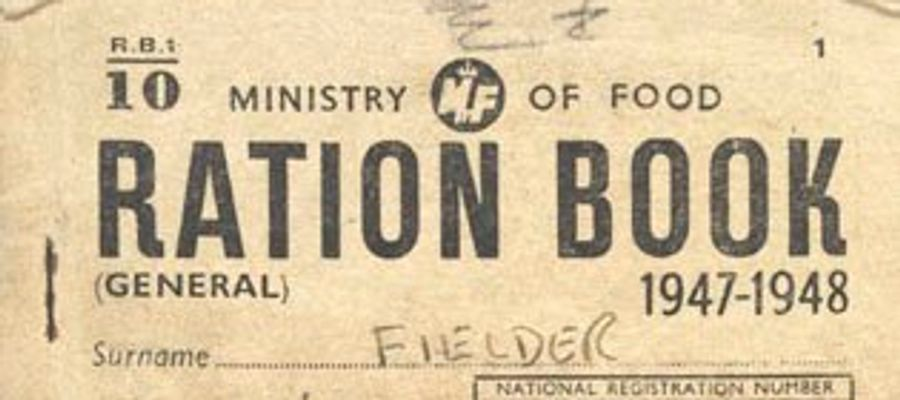# The WW2 Rationing Quiz

## Can you correctly identify if an item was rationed or not during the Second World War (1939-1945)?### Quiz Questions

• 1.
Potatoes
• A.
Rationed
• B.
Not Rationed
• 2.
Sugar
• A.
Rationed
• B.
Not rationed
• 3.
Cheese
• A.
Rationed
• B.
Not Rationed
• 4.
Carrots
• A.
Rationed
• B.
Not rationed
• 5.
Eggs
• A.
Rationed
• B.
Not rationed
• 6.
Chocolate
• A.
Rationed
• B.
Not rationed
• 7.
• A.
Rationed
• B.
Not rationed
• 8.
Beer
• A.
Rationed
• B.
Not rationed
• 9.
Meat
• A.
Rationed
• B.
Not rationed
• 10.
Soap
• A.
Rationed
• B.
Not rationed
• 11.
Broccoli
• A.
Rationed
• B.
Not rationed
• 12.
Blackberries
• A.
Rationed
• B.
Not rationed
• 13.
Tea
• A.
Rationed
• B.
Not rationed
• 14.
Fish
• A.
Rationed
• B.
Not rationed
• 15.
Milk
• A.
Rationed
• B.
Not rationed

### Quiz Outcomes

• 1.
Now you're an expert on WW2 rationing!